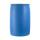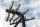# Quadratic equation + Pythagorean theorem - examples

1. Find parametersFind parameters of the circle in the plane - coordinates of center and radius: ?
2. Trapezoid MOThe rectangular trapezoid ABCD with right angle at point B, |AC| = 12, |CD| = 8, diagonals are perpendicular to each other. Calculate the perimeter and area of ​​the trapezoid.
3. CuboidCuboid with edge a=16 cm and body diagonal u=45 cm has volume V=11840 cm3. Calculate the length of the other edges.
4. MO SK/CZ Z9–I–3John had the ball that rolled into the pool and it swam in the water. Its highest point was 2 cm above the surface. Diameter of circle that marked the water level on the surface of the ball was 8 cm. Determine the diameter of John ball.
5. RightDetermine angles of the right triangle with the hypotenuse c and legs a, b, if: ?
6. Right triangleLegs of right are in ratio a:b = 6:8. Hypotenuse has a length of 61 cm. Calculate the perimeter and area of the triangle.
7. Rhombus and inscribed circleIt is given a rhombus with side a = 6 cm and the radius of the inscribed circle r = 2 cm. Calculate the length of its two diagonals.
8. R triangleCalculate the area of a right triangle whose longer leg is 6 dm shorter than the hypotenuse and 3 dm longer than the shorter leg.
9. Rectangle SSPerimeter of a rectangle is 268 cm and its diagonal is 99.3 cm. Determine the dimensions of the rectangle.
10. CircleCircle touch two parallel lines p and q; and its center lies on a line a, which is secant of lines p and q. Write the equation of circle and determine the coordinates of the center and radius. p: x-10 = 0 q: -x-19 = 0 a: 9x-4y+5 = 0
11. Perimeter and legsDetermine the perimeter of a right triangle if the length of one leg is 75% length of the second leg and its content area is 24 cm2.
12. Sphere and coneWithin the sphere of radius G = 33 cm inscribe cone with largest volume. What is that volume and what are the dimensions of the cone?
13. Trapezoidtrapezoid ABCD a = 35 m, b=28 m c = 11 m and d = 14 m. How to calculate its area?
14. TankIn the middle of a cylindrical tank with a bottom diameter 251 cm is standing rod which is 13 cm above the water surface. If we bank rod its end reach surface of the water just by the tank wall. How deep is the tank?
15. Rectangle diagonalsIt is given rectangle with area 24 cm2 a circumference 20 cm. The length of one side is 2 cm larger than length of second side. Calculate the length of the diagonal. Length and width are yet expressed in natural numbers.
16. ColumnPerpendicular pole high 8 m tall broke and its toe fell 2.7 m from the bottom of the pole. At what height above the ground pole broke?
17. TriangleCalculate the sides of the triangle if its area S = 630 and the second cathethus is shorter by 17.
18. Euclid theoremsCalculate the sides of a right triangle if leg a = 6 cm and a section of the hypotenuse, which is located adjacent the second leg b is 5cm.
19. RhombusThe rhombus with area 68 has one diagonal is longer by 6 than second one. Calculate the length of the diagonals and rhombus sides.
20. 3d vector componentThe vector u = (3.9, u3) and the length of the vector u is 12. What is is u3?

Do you have an interesting mathematical example that you can't solve it? Enter it, and we can try to solve it.

To this e-mail address, we will reply solution; solved examples are also published here. Please enter e-mail correctly and check whether you don't have a full mailbox.

Looking for help with calculating roots of a quadratic equation? Pythagorean theorem is the base for the right triangle calculator.